# Selina Solutions Concise Maths Class 10 Chapter 13 Section and Mid-Point Formula Exercise 13(C)

In this exercise, students will be solving problems based on all the concepts of this chapter. For any assistance, the Selina Solutions for Class 10 Maths is the right place for all the references to solve problems and doubt clearances. Experts at BYJU’S have created the solutions as per the latest ICSE patterns and are easily available in PDF format too. The Concise Selina Solutions for Class 10 Maths Chapter 13 Section and Mid-Point Formula Exercise 13(C) PDF is available in the links attached below.

## Selina Solutions Concise Maths Class 10 Chapter 13 Section and Mid-Point Formula Exercise 13(C) Download PDF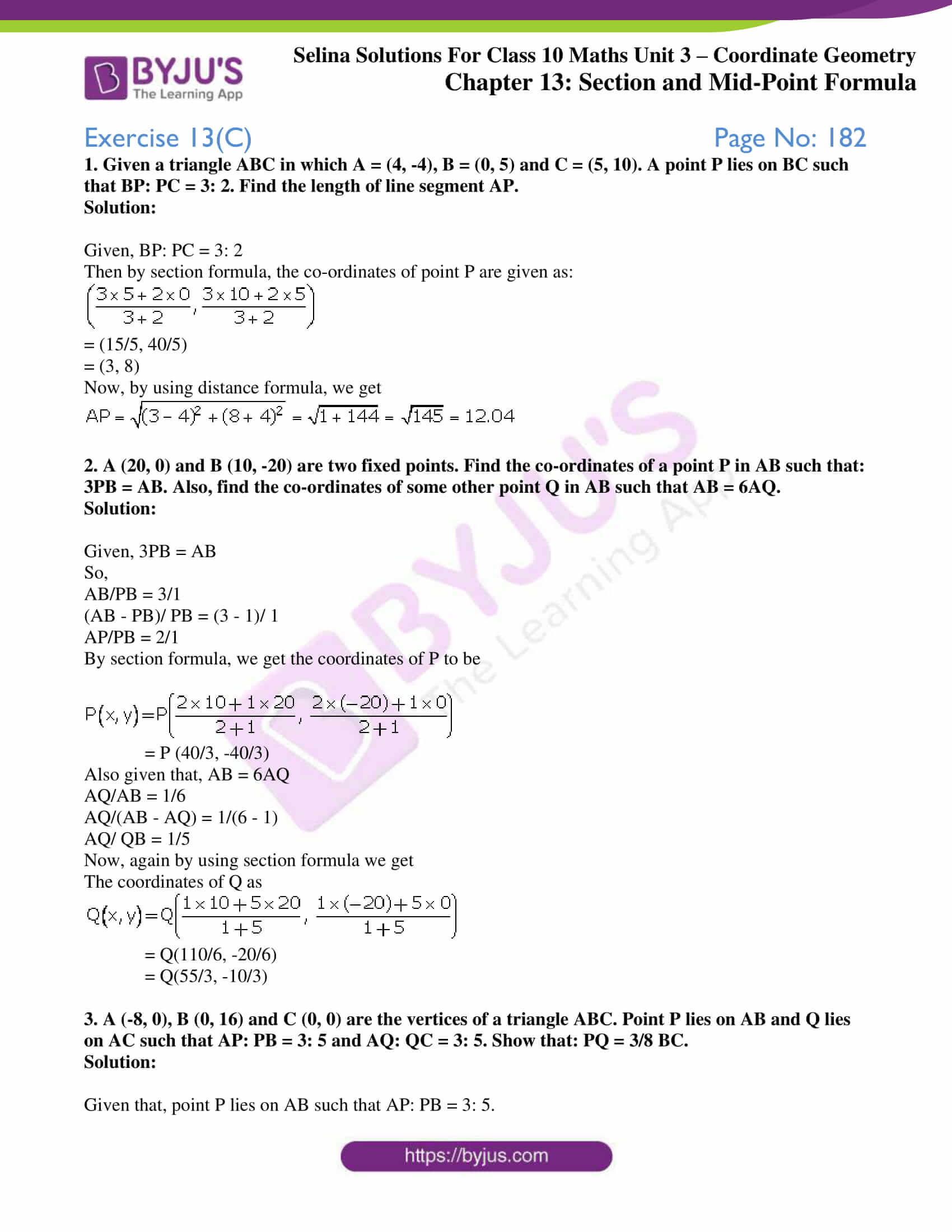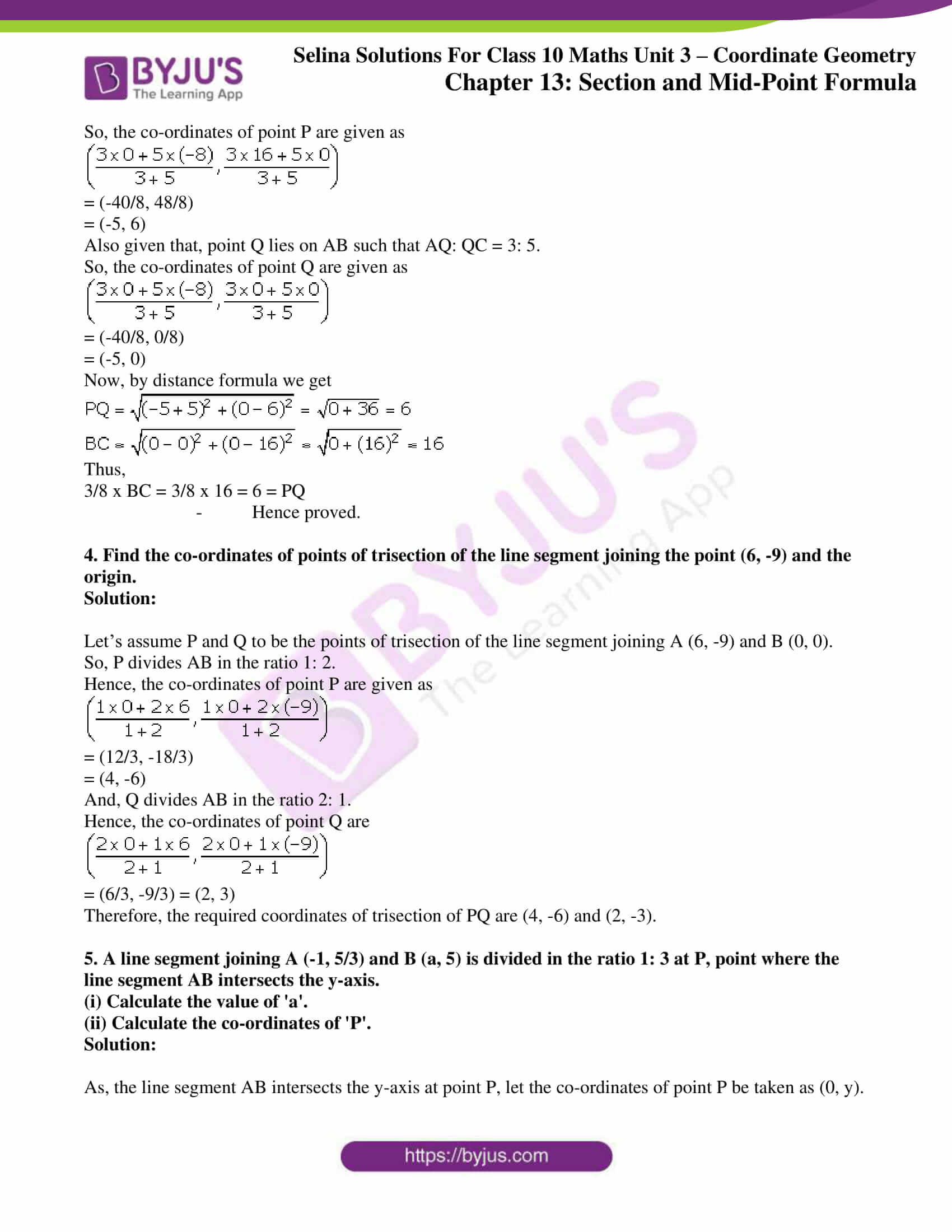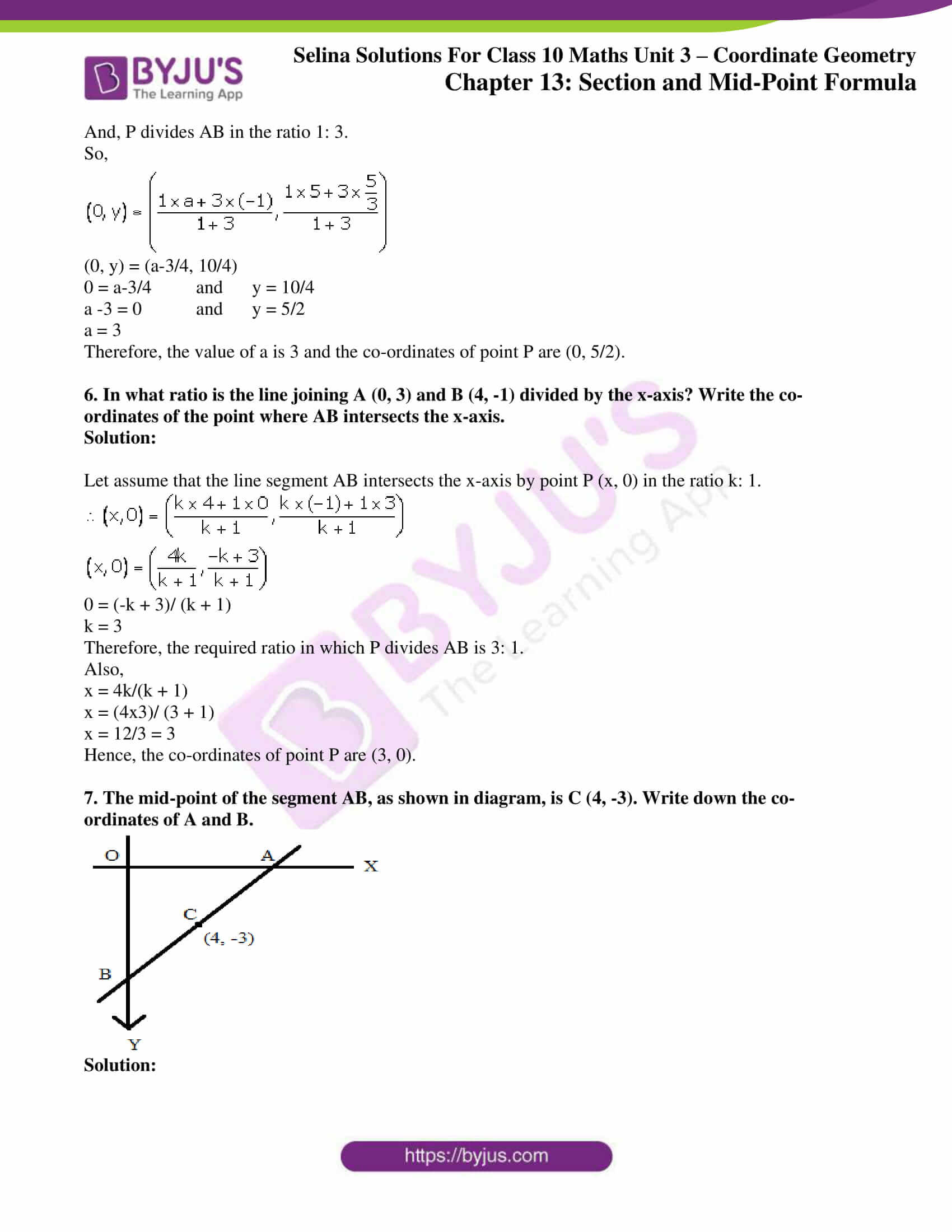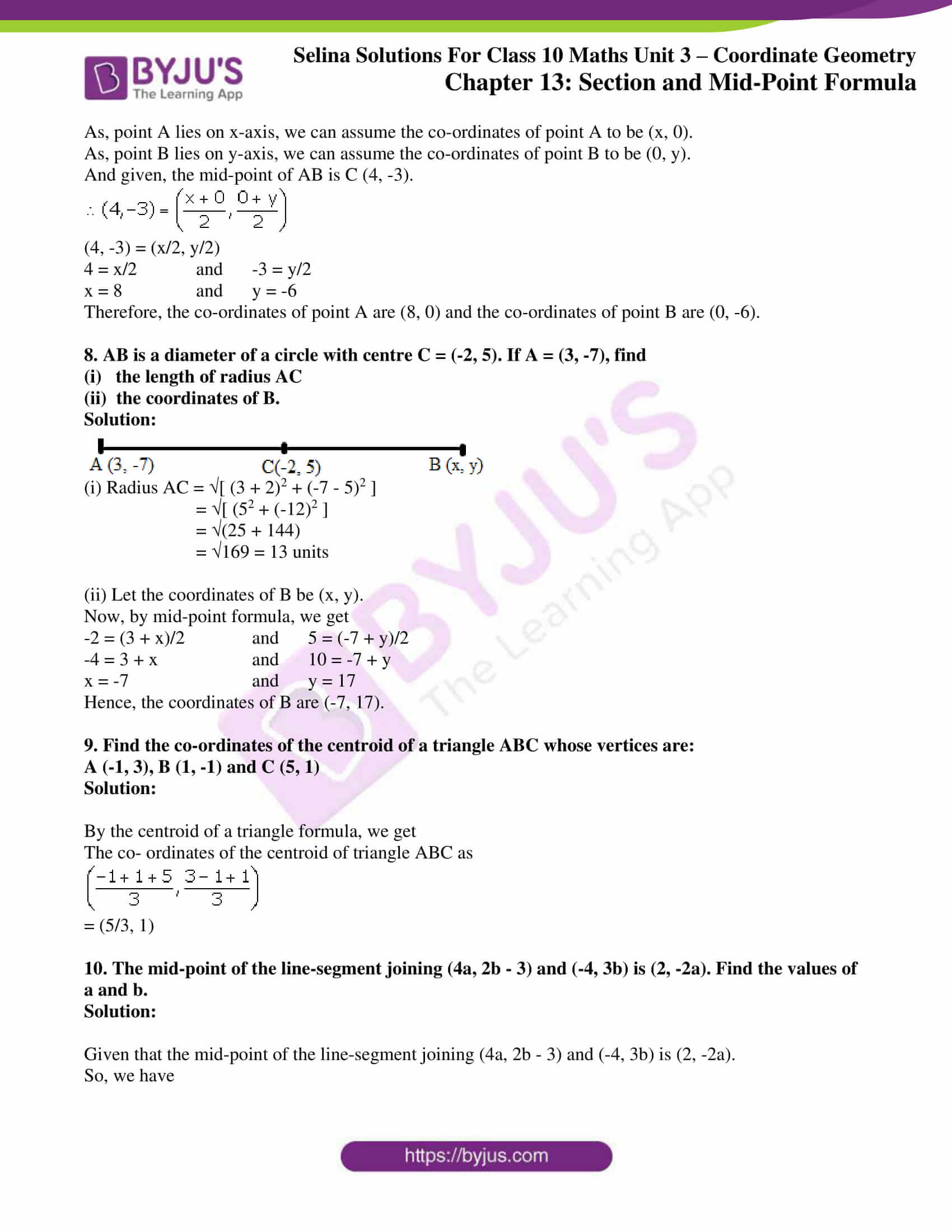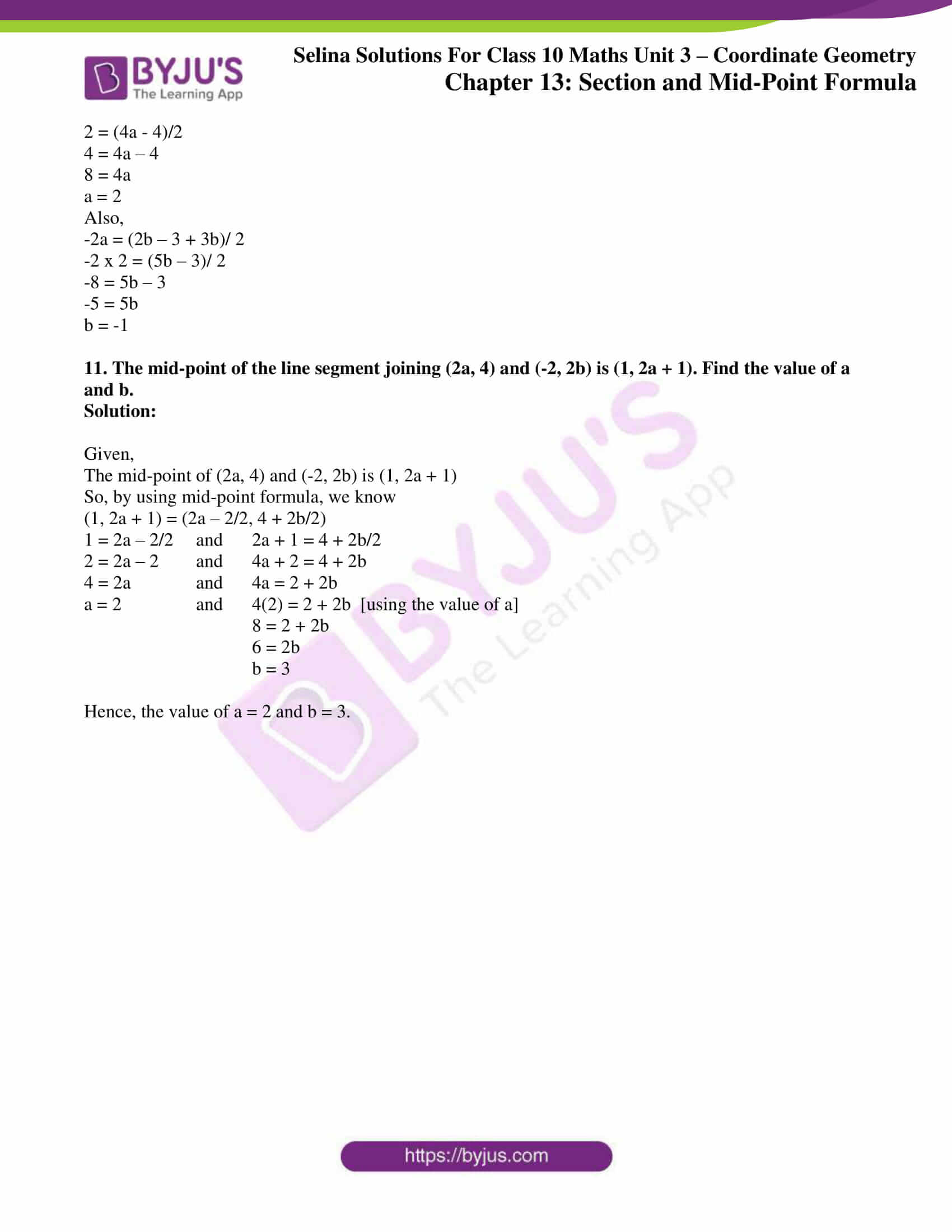### Access Selina Solutions Concise Maths Class 10 Chapter 13 Section and Mid-Point Formula Exercise 13(C)

1. Given a triangle ABC in which A = (4, -4), B = (0, 5) and C = (5, 10). A point P lies on BC such that BP: PC = 3: 2. Find the length of line segment AP.

Solution:

Given, BP: PC = 3: 2

Then by section formula, the co-ordinates of point P are given as:= (15/5, 40/5)

= (3, 8)

Now, by using distance formula, we get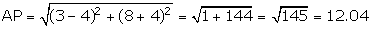2. A (20, 0) and B (10, -20) are two fixed points. Find the co-ordinates of a point P in AB such that: 3PB = AB. Also, find the co-ordinates of some other point Q in AB such that AB = 6AQ.

Solution:

Given, 3PB = AB

So,

AB/PB = 3/1

(AB – PB)/ PB = (3 – 1)/ 1

AP/PB = 2/1

By section formula, we get the coordinates of P to be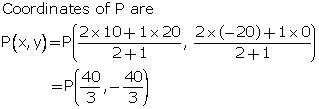= P (40/3, -40/3)

Also given that, AB = 6AQ

AQ/AB = 1/6

AQ/(AB – AQ) = 1/(6 – 1)

AQ/ QB = 1/5

Now, again by using section formula we get

The coordinates of Q as= Q(110/6, -20/6)

= Q(55/3, -10/3)

3. A (-8, 0), B (0, 16) and C (0, 0) are the vertices of a triangle ABC. Point P lies on AB and Q lies on AC such that AP: PB = 3: 5 and AQ: QC = 3: 5. Show that: PQ = 3/8 BC.

Solution:

Given that, point P lies on AB such that AP: PB = 3: 5.

So, the co-ordinates of point P are given as= (-40/8, 48/8)

= (-5, 6)

Also given that, point Q lies on AB such that AQ: QC = 3: 5.

So, the co-ordinates of point Q are given as= (-40/8, 0/8)

= (-5, 0)

Now, by distance formula we getThus,

3/8 x BC = 3/8 x 16 = 6 = PQ

– Hence proved.

4. Find the co-ordinates of points of trisection of the line segment joining the point (6, -9) and the origin.

Solution:

Let’s assume P and Q to be the points of trisection of the line segment joining A (6, -9) and B (0, 0).

So, P divides AB in the ratio 1: 2.

Hence, the co-ordinates of point P are given as= (12/3, -18/3)

= (4, -6)

And, Q divides AB in the ratio 2: 1.

Hence, the co-ordinates of point Q are= (6/3, -9/3) = (2, 3)

Therefore, the required coordinates of trisection of PQ are (4, -6) and (2, -3).

5. A line segment joining A (-1, 5/3) and B (a, 5) is divided in the ratio 1: 3 at P, point where the line segment AB intersects the y-axis.

(i) Calculate the value of ‘a’.

(ii) Calculate the co-ordinates of ‘P’.

Solution:

As, the line segment AB intersects the y-axis at point P, let the co-ordinates of point P be taken as (0, y).

And, P divides AB in the ratio 1: 3.

So,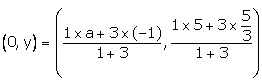(0, y) = (a-3/4, 10/4)

0 = a-3/4 and y = 10/4

a -3 = 0 and y = 5/2

a = 3

Therefore, the value of a is 3 and the co-ordinates of point P are (0, 5/2).

6. In what ratio is the line joining A (0, 3) and B (4, -1) divided by the x-axis? Write the co-ordinates of the point where AB intersects the x-axis.

Solution:

Let assume that the line segment AB intersects the x-axis by point P (x, 0) in the ratio k: 1.0 = (-k + 3)/ (k + 1)

k = 3

Therefore, the required ratio in which P divides AB is 3: 1.

Also,

x = 4k/(k + 1)

x = (4×3)/ (3 + 1)

x = 12/3 = 3

Hence, the co-ordinates of point P are (3, 0).

7. The mid-point of the segment AB, as shown in diagram, is C (4, -3). Write down the co-ordinates of A and B.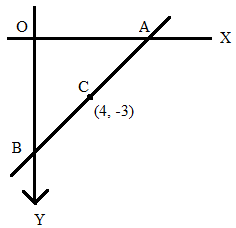Solution:

As, point A lies on x-axis, we can assume the co-ordinates of point A to be (x, 0).

As, point B lies on y-axis, we can assume the co-ordinates of point B to be (0, y).

And given, the mid-point of AB is C (4, -3).(4, -3) = (x/2, y/2)

4 = x/2 and -3 = y/2

x = 8 and y = -6

Therefore, the co-ordinates of point A are (8, 0) and the co-ordinates of point B are (0, -6).

8. AB is a diameter of a circle with centre C = (-2, 5). If A = (3, -7), find

(i)   the length of radius AC

(ii)  the coordinates of B.

Solution: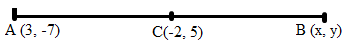(i) Radius AC = √[ (3 + 2)2 + (-7 – 5)2 ]

= √[ (52 + (-12)2 ]

= √(25 + 144)

= √169 = 13 units

(ii) Let the coordinates of B be (x, y).

Now, by mid-point formula, we get

-2 = (3 + x)/2 and 5 = (-7 + y)/2

-4 = 3 + x and 10 = -7 + y

x = -7 and y = 17

Hence, the coordinates of B are (-7, 17).

9. Find the co-ordinates of the centroid of a triangle ABC whose vertices are:

A (-1, 3), B (1, -1) and C (5, 1)

Solution:

By the centroid of a triangle formula, we get

The co- ordinates of the centroid of triangle ABC as= (5/3, 1)

10. The mid-point of the line-segment joining (4a, 2b – 3) and (-4, 3b) is (2, -2a). Find the values of a and b.

Solution:

Given that the mid-point of the line-segment joining (4a, 2b – 3) and (-4, 3b) is (2, -2a).

So, we have

2 = (4a – 4)/2

4 = 4a – 4

8 = 4a

a = 2

Also,

-2a = (2b – 3 + 3b)/ 2

-2 x 2 = (5b – 3)/ 2

-8 = 5b – 3

-5 = 5b

b = -1

11. The mid-point of the line segment joining (2a, 4) and (-2, 2b) is (1, 2a + 1). Find the value of a and b.

Solution:

Given,

The mid-point of (2a, 4) and (-2, 2b) is (1, 2a + 1)

So, by using mid-point formula, we know

(1, 2a + 1) = (2a – 2/2, 4 + 2b/2)

1 = 2a – 2/2 and 2a + 1 = 4 + 2b/2

2 = 2a – 2 and 4a + 2 = 4 + 2b

4 = 2a and 4a = 2 + 2b

a = 2 and 4(2) = 2 + 2b [using the value of a]

8 = 2 + 2b

6 = 2b

b = 3

Hence, the value of a = 2 and b = 3.